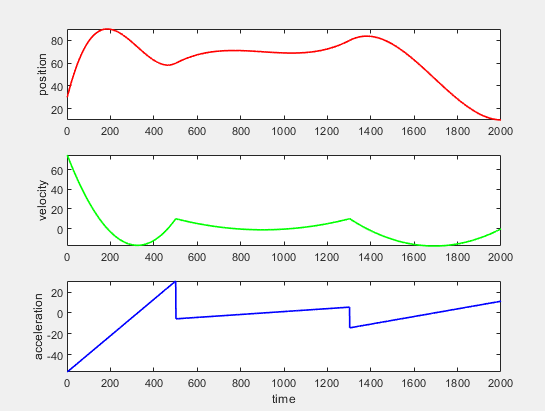# （一）三次多项式轨迹规划

### 二、轨迹规划中的三次多项式

x ( t ) = c 0 + c 1 t + c 2 t 2 + c 3 t 3 v ( t ) = c 1 + 2 c 2 t + 3 c 3 t 2 a ( t ) = 2 c 2 + 6 c 3 t \begin{aligned} &x(t)=c_0+c_1t+c_2t^2+c_3t^3\\ &v(t)=c_1+2c_2t+3c_3t^2\\ &a(t)=2c_2+6c_3t\\ \end{aligned}

• 起始绝对量
• 起始速度
• 结束绝对量
• 结束速度

x ( t s ) = c 0 + c 1 t s + c 2 t s 2 + c 3 t s 3 = x s x ( t e ) = c 0 + c 1 t e + c 2 t e 2 + c 3 t e 3 = x e v ( t s ) = c 1 + 2 c 2 t s + 3 c 3 t s 2 = v s v ( t e ) = c 1 + 2 c 2 t e + 3 c 3 t e 2 = v e \begin{aligned} &x(t_s)=c_0+c_1t_s+c_2t_s^2+c_3t_s^3=x_s\\ &x(t_e)=c_0+c_1t_e+c_2t_e^2+c_3t_e^3=x_e\\ &v(t_s)=c_1+2c_2t_s+3c_3t_s^2=vs\\ &v(t_e)=c_1+2c_2t_e+3c_3t_e^2=ve \end{aligned}

[ 1 t s t s 2 t s 3 1 t e t e 2 t e 3 0 1 2 t s 3 t s 2 0 1 2 t e 3 t e 2 ] \begin{bmatrix} 1 & ts & ts^2&ts^3 \\ 1 & te & te^2&te^3 \\ 0 & 1 & 2ts&3ts^2\\ 0&1&2te&3te^2 \end{bmatrix}

T C = P C = T − 1 P \begin{aligned} &TC=P\\ &C=T^{-1}P \end{aligned}

Matlab实现如下,连续进行了三段曲线连续轨迹规划：0-1，1-2，2-3

% 三次多项式插补
close all
clear;
clc;

% 0-1
t0=0; x0=30; v0=75;
t1=5; x1=60; v1=10;
[x01,v01,a01]=plan(t0,x0,v0,t1,x1,v1);

% 1-2
t2=13; x2=80; v2=10;
[x12,v12,a12]=plan(0,x1,v1,t2-t1,x2,v2);

% 2-3
t3=20; x3=10; v3=0;
[x23,v23,a23]=plan(0,x2,v2,t3-t2,x3,v3);

x=[x01 x12 x23];
v=[v01 v12 v23];
a=[a01 a12 a23];

figure(1);
subplot(3,1,1);
plot(x,'r','LineWidth',1.2);
axis([ t0*100 t3*100 -inf inf]);
ylabel('position')

subplot(3,1,2);
plot(v,'g','LineWidth',1.2)
axis([ t0*100 t3*100 -inf inf]);
ylabel('velocity')

subplot(3,1,3);
plot(a,'b','LineWidth',1.2);
axis([ t0*100 t3*100 -inf inf]);
ylabel('acceleration')
xlabel('time')

function [x,v,a]=plan(ts,start_x,start_v,te,end_x,end_v)
para=[start_x,end_x,start_v,end_v]';
Tran=[1,ts,ts^2,ts^3;1,te,te^2,te^3;0,1,2*ts,3*ts^2;0,1,2*te,3*te^2];
C=(inv(Tran))*para;
c0=C(1);
c1=C(2);
c2=C(3);
c3=C(4);
x=[];v=[];a=[];
for i=ts:0.01:te
t=i;
x=[x c0+c1*t+c2*t^2+c3*t^3];
v=[v c1+2*c2*t+3*c3*t^2];
a=[a 2*c2+6*c3*t];
end
end03-22552207-142万+
10-10
04-22
07-016502
02-091万+
09-092492
03-247755
11-121651